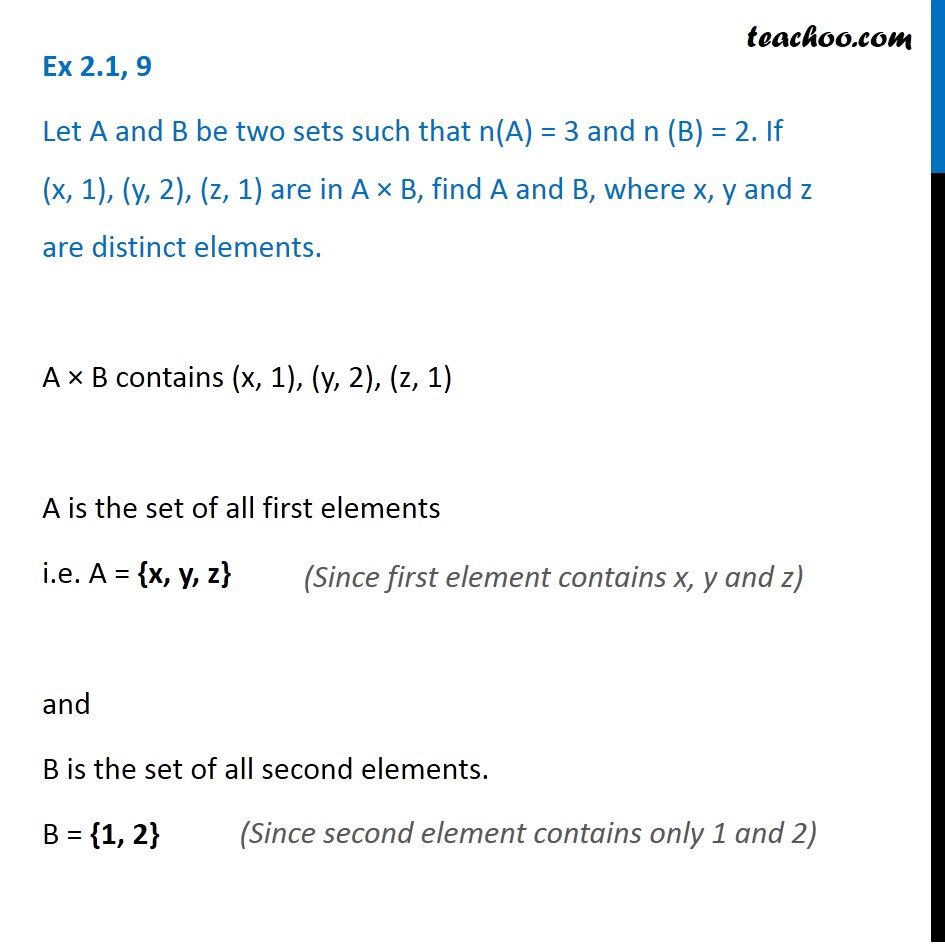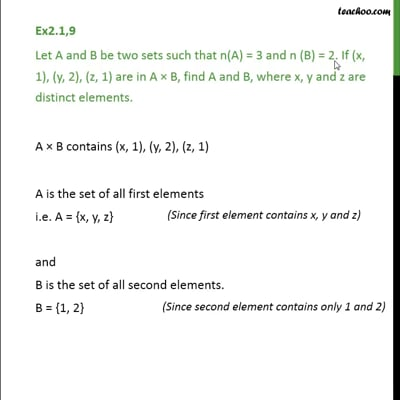Ex 2.1

Chapter 2 Class 11 Relations and Functions
Serial order wiseThis video is only available for Teachoo black users

Solve all your doubts with Teachoo Black (new monthly pack available now!)

### Transcript

Ex 2.1, 9 Let A and B be two sets such that n(A) = 3 and n (B) = 2. If (x, 1), (y, 2), (z, 1) are in A × B, find A and B, where x, y and z are distinct elements. A × B contains (x, 1), (y, 2), (z, 1) A is the set of all first elements i.e. A = {x, y, z} and B is the set of all second elements. B = {1, 2} (Since first element contains x, y and z) (Since second element contains only 1 and 2)